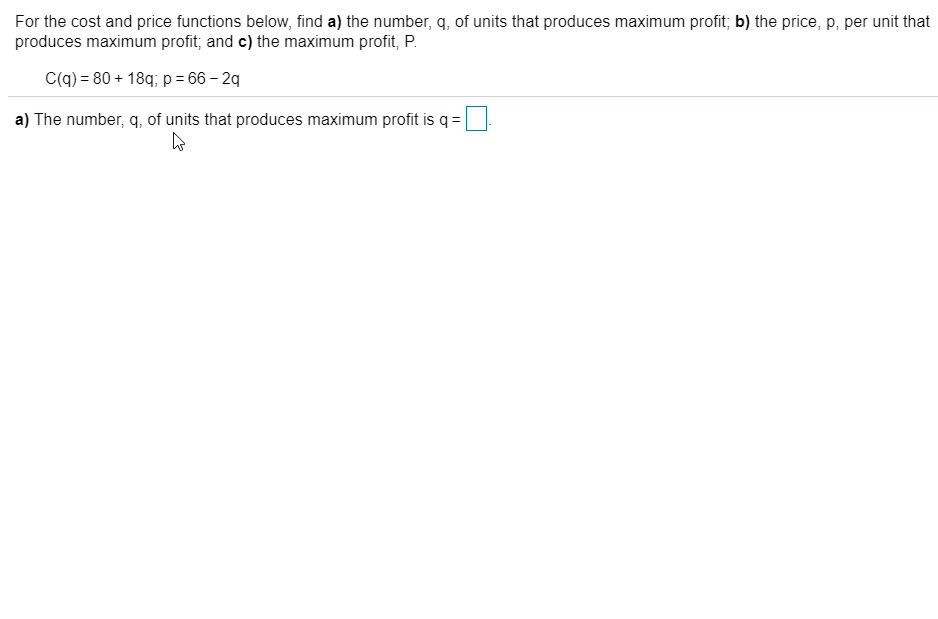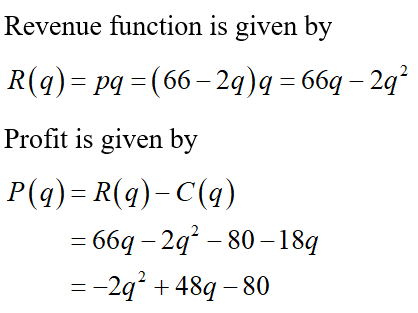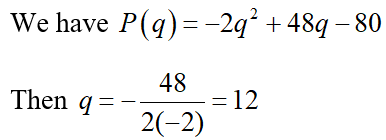# For the cost and price functions below, find a) the number, q, of units that produces maximum profit; b) the price, p, per unit thatproduces maximum profit; and c) the maximum profit, P.C(q) = 80 + 18q; p = 66 – 2qa) The number, q, of units that produces maximum profit is q =

Question
22 viewshelp_outlineImage TranscriptioncloseFor the cost and price functions below, find a) the number, q, of units that produces maximum profit; b) the price, p, per unit that produces maximum profit; and c) the maximum profit, P. C(q) = 80 + 18q; p = 66 – 2q a) The number, q, of units that produces maximum profit is q = fullscreen
check_circle

Step 1

Given

Cost function C(q) = 80 + 18q

Price function p = 66 – 2q

Step 2

Find the revenue and profit functionsStep 3

a) Clearly the above profit function is of the form of quadratic function f(x) = ax2 + bx + c and maximum occurs at the vertex point x = -b/2a.

Find the number of units that produces maximum profit.Therefore, the number, q, of units that produces maximum pro...

### Want to see the full answer?

See Solution

#### Want to see this answer and more?

Solutions are written by subject experts who are available 24/7. Questions are typically answered within 1 hour.*

See Solution
*Response times may vary by subject and question.
Tagged in